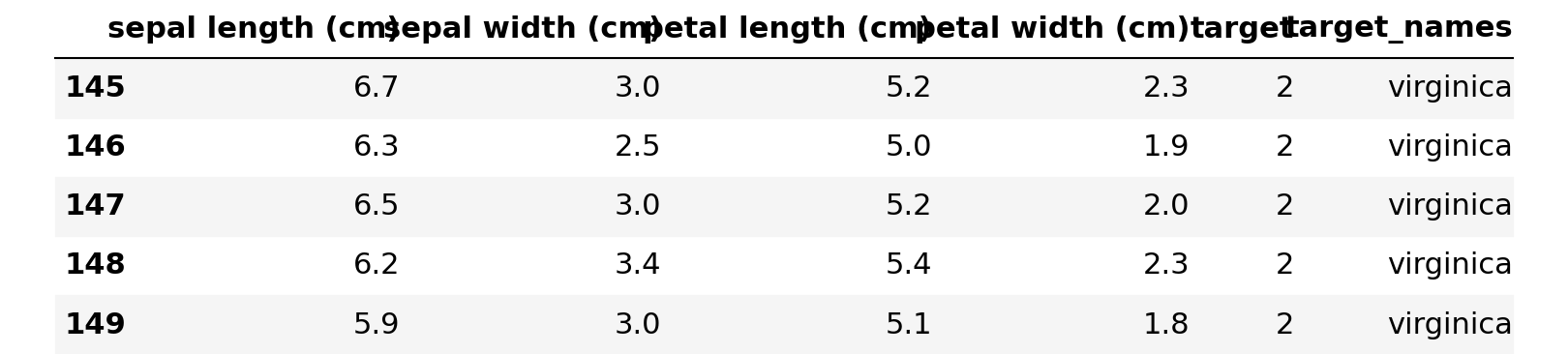# Algorithms from scratch: Support Vector MachineThis post was originally published by Kurtis Pykes at Towards Data Science

## Detailing and Building a Support Vector Machine from Scratch

A popular algorithm that is capable of performing linear or non-linear classification and regression, Support Vector Machines were the talk of the town before the rise of deep learning due to the exciting kernel trick — If the terminology makes no sense to you right now don’t worry about it. By the end of this post you’ll have an good understanding about the intuition of SVMs, what is happening under the hood of linear SVMs, and how to implement one in Python.

To see the full Algorithms from Scratch Series click on the link below.

## Intuition

In classification problems the objective of the SVM is to fit the largest possible margin between the 2 classes. On the contrary, regression task flips the objective of classification task and attempts to fit as many instances as possible within the margin — We will first focus on classification.

If we focus solely on the extremes of the data (the observations that are on the edges of the cluster) and we define a threshold to be the mid-point between the two extremes, we are left with a margin that we use to sepereate the two classes — this is often referred to as a hyperplane. When we apply a threshold that gives us the largest margin (meaning that we are strict to ensure that no instances land within the margin) to make classifications this is called Hard Margin Classification (some text refer to this as Maximal Margin Classification).

When detailing hard margin classification it always helps to see what is happening visually, hence Figure 2 is an example of a hard margin classification. To do this we will use the iris dataset from scikit-learn and utility function `plot_svm()` which you can find when you access the full code on github — link below.

## kurtispykes/ml-from-scratch

### Permalink Dismiss GitHub is home to over 50 million developers working together to host and review code, manage…

#### github.com

Note: This story was written straight from jupyter notebooks using python package `jupyter_to_medium` — for more information on this package click here — and the committed version on github is a first draft hence you may notice some alterations to this post.

# convert to DataFrame
df = pd.DataFrame(data=iris.data,
columns= iris.feature_names)

# store mapping of targets and target names
target_dict = dict(zip(set(iris.target), iris.target_names))

# add the target labels and the feature names
df[“target”] = iris.target
df[“target_names”] = df.target.map(target_dict)

# view the data
df.tail()Figure 1: Original Dataset

# fit the model with hard margin (Large C parameter)
svc = LinearSVC(loss=”hinge”, C=1000)
svc.fit(X, y)

plot_svm()Figure 2: Visualizing the decision boundary by SVM

Figure 2 displays how the Linear SVM uses hard margin classification to ensure that no instances falls within the margin. Although this looks good for our current scenario, we must be careful to take into account the pitfalls that come with performing hard margin classification:

1. Very sensitive to outliers
2. It only works when the classes are linearly separable